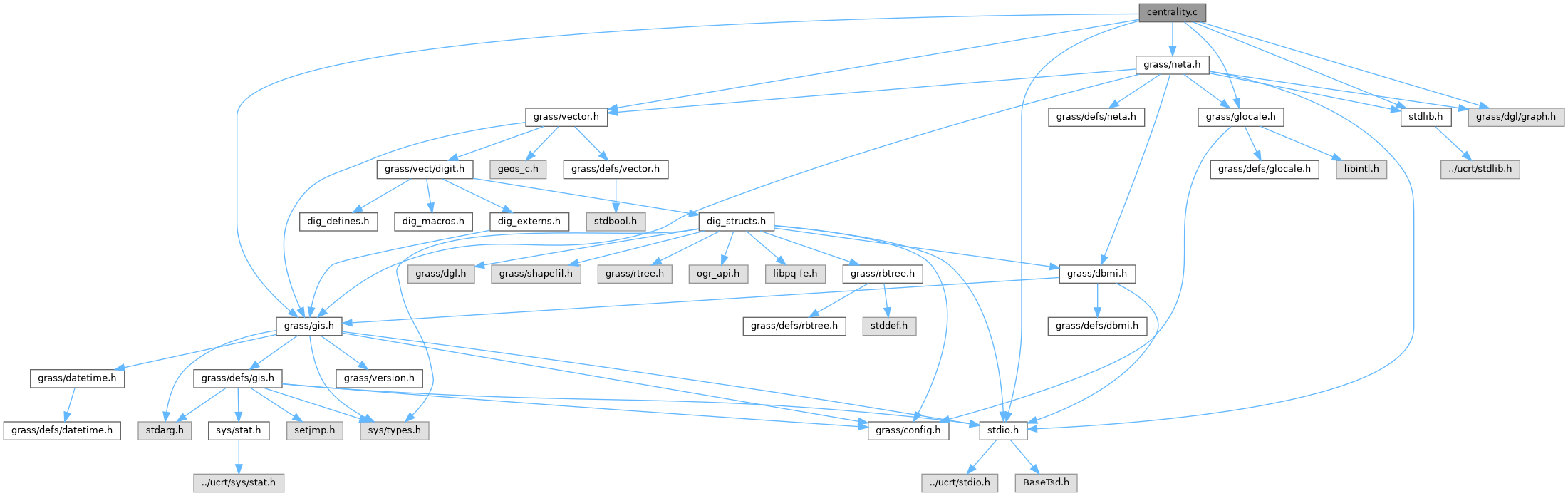GRASS GIS 8 Programmer's Manual  8.2.2dev(2023)-5c00b4e944
centrality.c File Reference

Network Analysis library - centrality. More...

`#include <stdio.h>`
`#include <stdlib.h>`
`#include <grass/gis.h>`
`#include <grass/vector.h>`
`#include <grass/glocale.h>`
`#include <grass/dgl/graph.h>`
`#include <grass/neta.h>`
Include dependency graph for centrality.c:Go to the source code of this file.

## Functions

void NetA_degree_centrality (dglGraph_s *graph, double *degree)
Computes degree centrality measure. More...

int NetA_eigenvector_centrality (dglGraph_s *graph, int iterations, double error, double *eigenvector)
Computes eigenvector centrality using edge costs as weights. More...

int NetA_betweenness_closeness (dglGraph_s *graph, double *betweenness, double *closeness)
Computes betweenness and closeness centrality measure using Brandes algorithm. More...

## Detailed Description

Network Analysis library - centrality.

Centrality measures

(C) 2009-2010 by Daniel Bundala, and the GRASS Development Team

This program is free software under the GNU General Public License (>=v2). Read the file COPYING that comes with GRASS for details.

Definition in file centrality.c.

## ◆ NetA_betweenness_closeness()

 int NetA_betweenness_closeness ( dglGraph_s * graph, double * betweenness, double * closeness )

Computes betweenness and closeness centrality measure using Brandes algorithm.

Edge costs must be nonnegative. If some edge costs are negative then the behaviour of this method is undefined.

Parameters
 graph input graph [out] betweenness betweeness values [out] closeness cloneness values
Returns
0 on success
-1 on failure

Definition at line 126 of file centrality.c.

## ◆ NetA_degree_centrality()

 void NetA_degree_centrality ( dglGraph_s * graph, double * degree )

Computes degree centrality measure.

Array degree has to be properly initialised to nnodes+1 elements

Parameters
 graph input graph [out] degree array of degrees

Definition at line 32 of file centrality.c.

References dglGet_NodeCount(), dglGetNode(), and dglNodeGet_OutDegree().

## ◆ NetA_eigenvector_centrality()

 int NetA_eigenvector_centrality ( dglGraph_s * graph, int iterations, double error, double * eigenvector )

Computes eigenvector centrality using edge costs as weights.

Parameters
 graph input graph iterations number of iterations error ? [out] eigenvector eigen vector value
Returns
0 on success
-1 on failure

Definition at line 54 of file centrality.c.